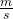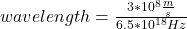## The frequency of an X ray is 6.5 x 1018 Hz. What is the X ray’s wavelength? .

Question

The frequency of an X ray is 6.5 x 1018 Hz. What is the X ray’s wavelength? .

in progress 0
6 months 2021-07-21T12:46:02+00:00 1 Answers 30 views 0

The X ray’s wavelength is 4.61*10⁻¹¹ m

Explanation:

Wavelength is the minimum distance between two successive points on the wave that are in the same state of vibration. It is expressed in units of length (m).

Frequency is the number of vibrations that occur in a unit of time. Its unit is s⁻¹ or hertz (Hz).

The propagation speed is the speed with which the wave propagates in the medium, that is, it is the magnitude that measures the speed at which the wave’s disturbance propagates along its displacement. Relate the wavelength (λ) and the frequency (f) inversely proportional using the following equation:

v = f * λ.

In this case:

• The frecuency f= 6.5*10¹⁸ Hz
• The speed v=c= 3*10⁸Replacing:

3*10⁸= 6.5*10¹⁸ Hz* λ

Solving:λ= 4.61*10⁻¹¹ m

The X ray’s wavelength is 4.61*10⁻¹¹ m